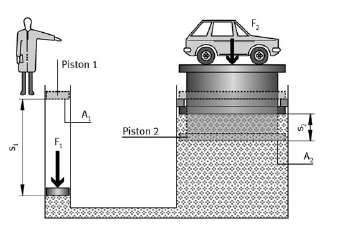﻿ Displacement transmission – Hydraulic Schematic Troubleshooting

## Displacement transmission

If a load F2 is to be lifted a distance S2 in line with the principle described above, the piston P1 must displace a specific quantity of liquid which lifts the piston P2 by a distance S2Displacement transmission

The necessary displacement volume is calculated as follows:
V1 = S1 · A1 and V2 = S2 · T2
Since the displacement volumes are identical (V1 = V2), the following equation is valid:
s1 · A1 = s2 · A2
From this it can be seen that the distance s1 must be greater than the distance s2 since the area A1 is smaller than the area A2.

The displacement of the piston is in inverse ratio to its area. This law can be used to calculate the values s1 and s2. For example, for s2 and A1.